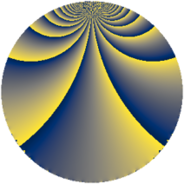# Properties

 Label 385.2.kLevel $385$ Weight $2$ Character orbit 385.k Rep. character $\chi_{385}(43,\cdot)$ Character field $\Q(\zeta_{4})$ Dimension $72$ Newform subspaces $1$ Sturm bound $96$ Trace bound $0$

# Related objects

## Defining parameters

 Level: $$N$$ $$=$$ $$385 = 5 \cdot 7 \cdot 11$$ Weight: $$k$$ $$=$$ $$2$$ Character orbit: $$[\chi]$$ $$=$$ 385.k (of order $$4$$ and degree $$2$$) Character conductor: $$\operatorname{cond}(\chi)$$ $$=$$ $$55$$ Character field: $$\Q(i)$$ Newform subspaces: $$1$$ Sturm bound: $$96$$ Trace bound: $$0$$

## Dimensions

The following table gives the dimensions of various subspaces of $$M_{2}(385, [\chi])$$.

Total New Old
Modular forms 104 72 32
Cusp forms 88 72 16
Eisenstein series 16 0 16

## Trace form

 $$72q + 4q^{3} + O(q^{10})$$ $$72q + 4q^{3} - 4q^{11} + 16q^{12} + 16q^{15} - 72q^{16} - 24q^{20} + 44q^{22} - 4q^{23} - 28q^{25} + 64q^{26} + 4q^{27} - 32q^{31} + 32q^{33} - 104q^{36} + 4q^{37} - 104q^{38} + 12q^{45} - 16q^{47} + 24q^{48} - 16q^{53} - 32q^{55} + 48q^{56} - 32q^{58} + 200q^{60} + 64q^{66} - 68q^{67} + 16q^{70} + 72q^{71} - 48q^{75} + 8q^{77} - 56q^{78} + 80q^{80} - 80q^{81} - 104q^{82} - 80q^{86} + 32q^{88} - 24q^{91} - 136q^{92} - 68q^{93} - 44q^{97} + O(q^{100})$$

## Decomposition of $$S_{2}^{\mathrm{new}}(385, [\chi])$$ into newform subspaces

Label Dim. $$A$$ Field CM Traces $q$-expansion
$$a_2$$ $$a_3$$ $$a_5$$ $$a_7$$
385.2.k.a $$72$$ $$3.074$$ None $$0$$ $$4$$ $$0$$ $$0$$

## Decomposition of $$S_{2}^{\mathrm{old}}(385, [\chi])$$ into lower level spaces

$$S_{2}^{\mathrm{old}}(385, [\chi]) \cong$$ $$S_{2}^{\mathrm{new}}(55, [\chi])$$$$^{\oplus 2}$$